Create a new printableGeometry
Math Worksheets

Sample - Click above to make a new math worksheet (PDF).
 Name _____________________________Date ___________________
Prisms and Cylinders
Find the volume of each solid to the nearest tenth. (use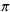= 3.14)

1.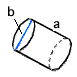a  = 4 ft b  = 65 ft

2.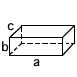a  = 55 km b  = 17.7 km c  = 14.1 km

3.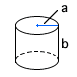a  = 8 m b  = 12 m

 4 * This is a pre-made sheet.Use the link at the top of the page for a printable page.
5.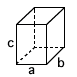a  = 16.8 yd b  = 12.9 yd c  = 39.1 yd

6.a  = 38 in b  = 60 in

7.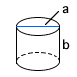a  = 9 cm b  = 23 cm

8.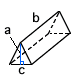a  = 8.18 in b  = 14 in c  = 14 in

9.a  = 3 m b  = 19 m

10.a  = 27 ft b  = 55 ft

11.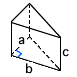a  = 7.31 yd b  = 4 yd c  = 13 yd

12.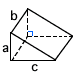a  = 3.46 km b  = 2 km c  = 12 km